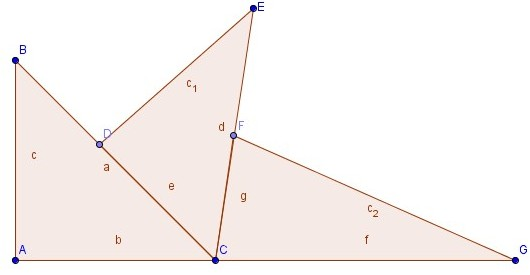# The three wedges of Po

Geometry Level 3In the figure above, there are three perpendicular wedges as shown where $\angle A=\angle D=\angle F= 90°.$ Further $AC=DC=FC=P$ and $AB=x, DE=y, FG=z.$ The points A, C & G are collinear. Find the value of $P^2 \left(\frac{1}{xy}+\frac{1}{yz}+\frac{1}{zx} \right).$

×